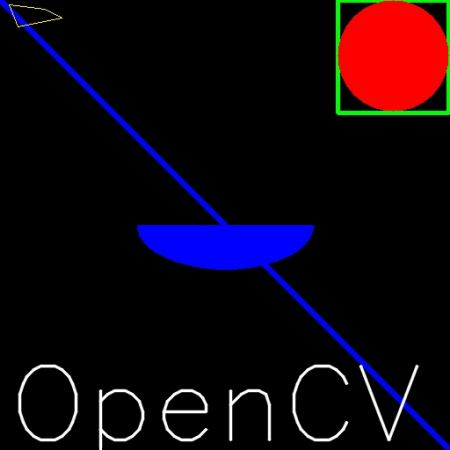# Drawing Functions in OpenCV¶

## Goal¶

• Learn to draw different geometric shapes with OpenCV
• You will learn these functions : cv2.line(), cv2.circle() , cv2.rectangle(), cv2.ellipse(), cv2.putText() etc.

## Code¶

In all the above functions, you will see some common arguments as given below:

• img : The image where you want to draw the shapes
• color : Color of the shape. for BGR, pass it as a tuple, eg: `(255,0,0)` for blue. For grayscale, just pass the scalar value.
• thickness : Thickness of the line or circle etc. If -1 is passed for closed figures like circles, it will fill the shape. default thickness = 1
• lineType : Type of line, whether 8-connected, anti-aliased line etc. By default, it is 8-connected. `cv2.LINE_AA` gives anti-aliased line which looks great for curves.

### Drawing Line¶

To draw a line, you need to pass starting and ending coordinates of line. We will create a black image and draw a blue line on it from top-left to bottom-right corners.

```import numpy as np
import cv2

# Create a black image
img = np.zeros((512,512,3), np.uint8)

# Draw a diagonal blue line with thickness of 5 px
img = cv2.line(img,(0,0),(511,511),(255,0,0),5)
```

### Drawing Rectangle¶

To draw a rectangle, you need top-left corner and bottom-right corner of rectangle. This time we will draw a green rectangle at the top-right corner of image.

```img = cv2.rectangle(img,(384,0),(510,128),(0,255,0),3)
```

### Drawing Circle¶

To draw a circle, you need its center coordinates and radius. We will draw a circle inside the rectangle drawn above.

```img = cv2.circle(img,(447,63), 63, (0,0,255), -1)
```

### Drawing Ellipse¶

To draw the ellipse, we need to pass several arguments. One argument is the center location (x,y). Next argument is axes lengths (major axis length, minor axis length). `angle` is the angle of rotation of ellipse in anti-clockwise direction. `startAngle` and `endAngle` denotes the starting and ending of ellipse arc measured in clockwise direction from major axis. i.e. giving values 0 and 360 gives the full ellipse. For more details, check the documentation of cv2.ellipse(). Below example draws a half ellipse at the center of the image.

```img = cv2.ellipse(img,(256,256),(100,50),0,0,180,255,-1)
```

### Drawing Polygon¶

To draw a polygon, first you need coordinates of vertices. Make those points into an array of shape `ROWSx1x2` where ROWS are number of vertices and it should be of type `int32`. Here we draw a small polygon of with four vertices in yellow color.

```pts = np.array([[10,5],[20,30],[70,20],[50,10]], np.int32)
pts = pts.reshape((-1,1,2))
img = cv2.polylines(img,[pts],True,(0,255,255))
```

Note

If third argument is `False`, you will get a polylines joining all the points, not a closed shape.

Note

`cv2.polylines()` can be used to draw multiple lines. Just create a list of all the lines you want to draw and pass it to the function. All lines will be drawn individually. It is more better and faster way to draw a group of lines than calling `cv2.line()` for each line.

To put texts in images, you need specify following things.
• Text data that you want to write
• Position coordinates of where you want put it (i.e. bottom-left corner where data starts).
• Font type (Check cv2.putText() docs for supported fonts)
• Font Scale (specifies the size of font)
• regular things like color, thickness, lineType etc. For better look, `lineType = cv2.LINE_AA` is recommended.

We will write OpenCV on our image in white color.

```font = cv2.FONT_HERSHEY_SIMPLEX
cv2.putText(img,'OpenCV',(10,500), font, 4,(255,255,255),2,cv2.LINE_AA)
```

### Result¶

So it is time to see the final result of our drawing. As you studied in previous articles, display the image to see it.